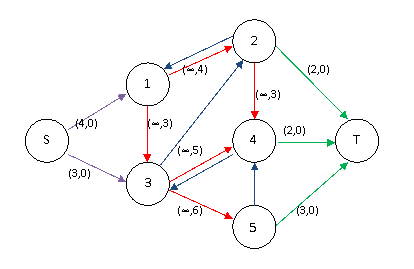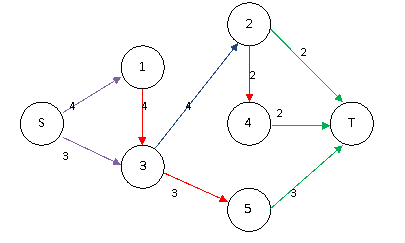# BZOJ-1061 志愿者招募 线性规划转最小费用最大流+数学模型 建模

本来一眼建模,以为傻逼题,然后发现自己傻逼...根本没想到神奇的数学模型.....


1061: [Noi2008]志愿者招募
Time Limit: 20 Sec Memory Limit: 162 MB
Submit: 3014 Solved: 1864
[Submit][Status][Discuss]

Description

Input

Output

Sample Input
3 3
2 3 4
1 2 2
2 3 5
3 3 2

Sample Output
14

HINT

SourceP = X + X >= 4
P = X + X >= 2
P = X + X +X >= 5
P = X >= 3

P = X + X - Y = 4
P = X + X - Y = 2
P = X + X +X - Y = 5
P = X - Y = 3

① P - P = X + X - Y = 4
② P - P = X - X -Y +Y = -2
③ P - P = X + X - X - Y + Y =3
④ P - P = - X - X + Y - Y = -2
⑤ P - P = - X + Y = -3① - X - X + Y + 4 = 0
② - X + X + Y - Y - 2 = 0
③ - X - X + X + Y - Y + 3 = 0
④ X + X - Y + Y - 2 = 0
⑤ X - Y - 3 = 0

(建模转自Beyond the Void,orz)

My code:

#include<iostream>
#include<cstdio>
#include<algorithm>
#include<cstring>
#include<cmath>
using namespace std;
{
int x=0,f=1;char ch=getchar();
while(ch<'0'||ch>'9'){if(ch=='-')f=-1;ch=getchar();}
while(ch>='0'&&ch<='9'){x*=10;x+=ch-'0';ch=getchar();}
return x*f;
}
#define inf 0x7fffffff
int n,m;
struct data{int to,next,cap,cost;}edge;
int ans=0;
int S,T;
bool visit;
int dis;
int q,h,t;
bool mark;

void add(int u,int v,int w,int c)
{
cnt++;
edge[cnt].to=v; edge[cnt].cost=c; edge[cnt].cap=w;
}
void insert(int u,int v,int w,int c)
{
}

bool spfa()
{
memset(visit,0,sizeof(visit));
for (int i=S; i<=T; i++) dis[i]=inf;
h=0,t=1;
q=T;dis[T]=0;visit[T]=1;
while (h<t)
{
int now=q[h];h++;
if (edge[i^1].cap && dis[now]-edge[i].cost<dis[edge[i].to])
{
dis[edge[i].to]=dis[now]-edge[i].cost;
if (!visit[edge[i].to])
{
q[t++]=edge[i].to;
visit[edge[i].to]=1;
}
}
visit[now]=0;
}
return dis[S]!=inf;
}

int dfs(int loc,int low)
{
mark[loc]=1;
if (loc==T) return low;
int w,used=0;
if (edge[i].cap && !mark[edge[i].to] && dis[edge[i].to]==dis[loc]-edge[i].cost)
{
w=dfs(edge[i].to,min(low-used,edge[i].cap));
ans+=w*edge[i].cost;
used+=w;
edge[i].cap-=w;edge[i^1].cap+=w;
if (used==low) return low;
}
return used;
}

void zkw()
{
int tmp=0;
while (spfa())
{
mark[T]=1;
while (mark[T])
{
memset(mark,0,sizeof(mark));
tmp+=dfs(S,inf);
}
}
}

void make()
{
S=0,T=1005;
int l=0,r;
for (int i=1; i<=n; i++)
{
int x=r-l;
if (x>0) insert(S,i,x,0);
else insert(i,T,-x,0);
insert(i+1,i,inf,0);
l=r;
}
insert(n+1,T,l,0);
for (int i=1; i<=m; i++)
{
insert(x,y+1,inf,c);
}
}

int main()
{
make();
zkw();
printf("%d\n",ans);
return 0;
}©️2019 CSDN 皮肤主题: 大白 设计师: CSDN官方博客